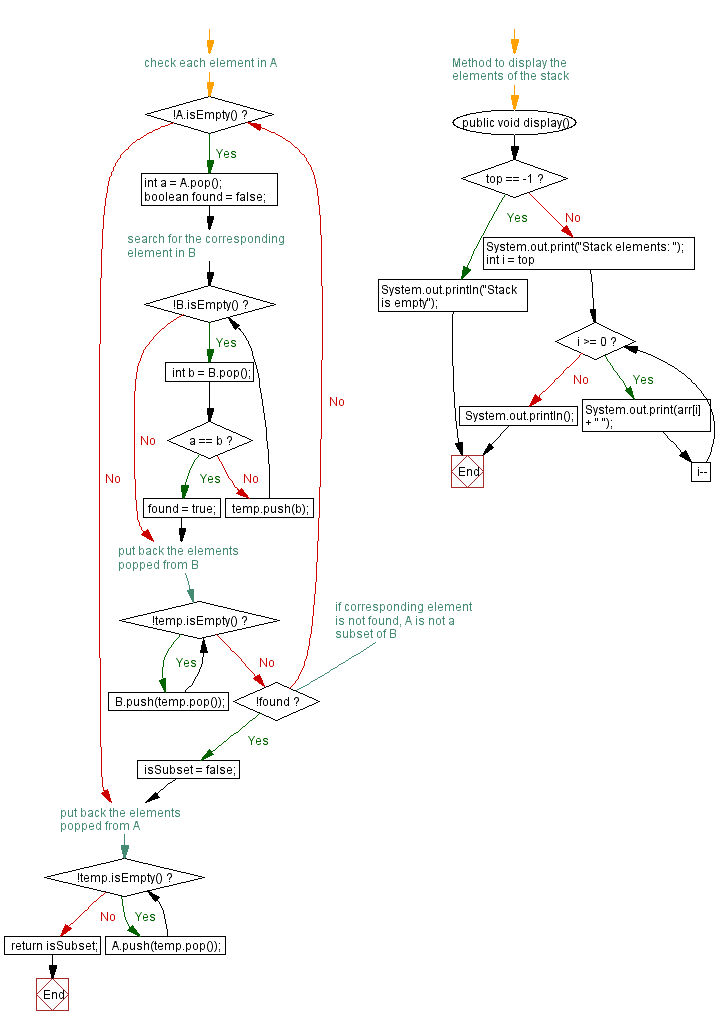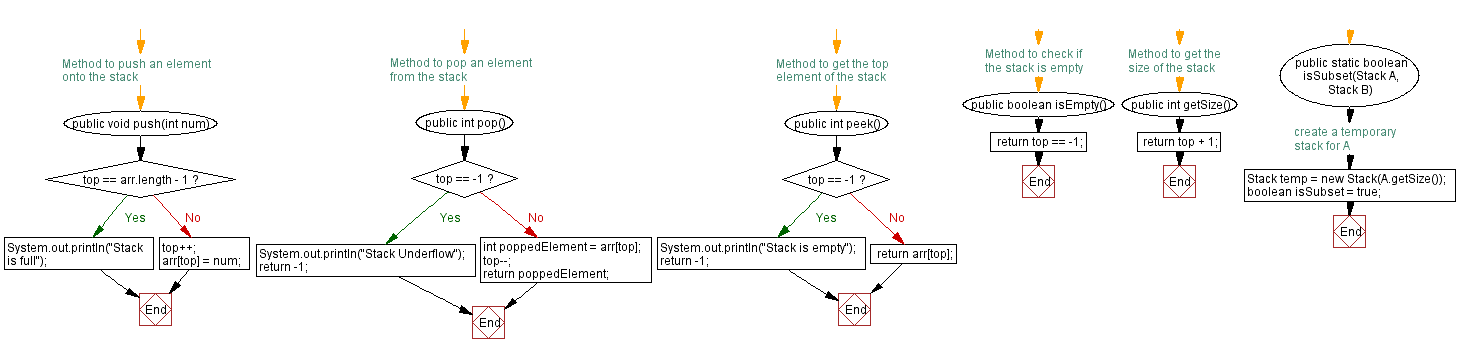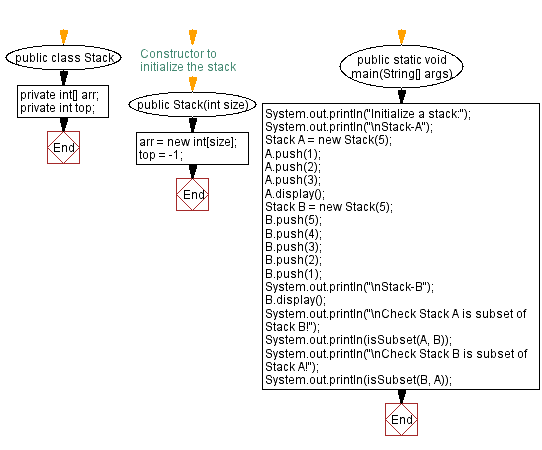# Java Exercises: Check if the stack is a subset of another stack

## Java Stack: Exercise-19 with Solution

Write a JavaScript program that implements a stack and checks if the stack is a subset of another stack.

Sample Solution:

Java Code:

``````import java.util.Scanner;

public class Stack {
private int[] arr;
private int top;

// Constructor to initialize the stack
public Stack(int size) {
arr = new int[size];
top = -1;
}

// Method to push an element onto the stack
public void push(int num) {
if (top == arr.length - 1) {
System.out.println("Stack is full");
} else {
top++;
arr[top] = num;
}
}

// Method to pop an element from the stack
public int pop() {
if (top == -1) {
System.out.println("Stack Underflow");
return -1;
} else {
int poppedElement = arr[top];
top--;
return poppedElement;
}
}

// Method to get the top element of the stack
public int peek() {
if (top == -1) {
System.out.println("Stack is empty");
return -1;
} else {
return arr[top];
}
}

// Method to check if the stack is empty
public boolean isEmpty() {
}

// Method to get the size of the stack
public int getSize() {
}

public static boolean isSubset(Stack A, Stack B) {
Stack temp = new Stack(A.getSize()); // create a temporary stack for A
boolean isSubset = true;

// check each element in A
while (!A.isEmpty()) {
int a = A.pop();
boolean found = false;

// search for the corresponding element in B
while (!B.isEmpty()) {
int b = B.pop();

if (a == b) {
found = true;
break;
} else {
temp.push(b);
}
}

// put back the elements popped from B
while (!temp.isEmpty()) {
B.push(temp.pop());
}

// if corresponding element is not found, A is not a subset of B
if (!found) {
isSubset = false;
break;
}
}

// put back the elements popped from A
while (!temp.isEmpty()) {
A.push(temp.pop());
}

return isSubset;
}

// Method to display the elements of the stack
public void display() {
if (top == -1) {
System.out.println("Stack is empty");
} else {
System.out.print("Stack elements: ");
for (int i = top; i >= 0; i--) {
System.out.print(arr[i] + " ");
}
System.out.println();
}
}

public static void main(String[] args) {
System.out.println("Initialize a stack:");
System.out.println("\nStack-A");
Stack A = new Stack(5);
A.push(1);
A.push(2);
A.push(3);
A.display();
Stack B = new Stack(5);
B.push(5);
B.push(4);
B.push(3);
B.push(2);
B.push(1);
System.out.println("\nStack-B");
B.display();
System.out.println("\nCheck Stack A is subset of Stack B!");
System.out.println(isSubset(A, B));
System.out.println("\nCheck Stack B is subset of Stack A!");
System.out.println(isSubset(B, A));
}
}
```
```

Sample Output:

```Initialize a stack:

Stack-A
Stack elements: 3 2 1

Stack-B
Stack elements: 1 2 3 4 5

Check Stack A is subset of Stack B!
true

Check Stack B is subset of Stack A!
false
```

Flowchart:Live Demo:

Java Code Editor:

Improve this sample solution and post your code through Disqus

Java Stack Previous: Merge two stacks into one.
Java Stack Exercises Next: Checks if two stacks are equal.

What is the difficulty level of this exercise?

Test your Programming skills with w3resource's quiz.

﻿